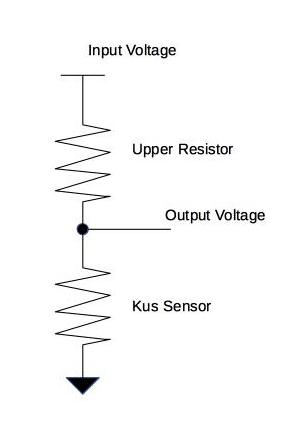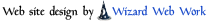Devices << VirtualDevices << ConversionDevices Software Overview  |  Sitemap  |  Downloads  |  Developers  |  ForumsHome Devices FAQs How-Tos Projects Tutorials Recipes

# Conversion Devices

Conversion Devices convert one type of unit to another. Conversion Devices are a specific type of Virtual Device. We support the following Conversion Devices.

Kus 240-33 Ohm Tank Sensor - converts voltage from a sensor in a fluid tank to fullness readings
RTD to Temperature Converter - converts resistances from RTD sensors to temperature

# Kus 240-33 Ohm Tank Sensor

The Kus 240-33 Ohm Tank Sensor Device converts voltages from a Kus 240-33 Ohm tank sensor circuit into tank fullness readings. The Kus Tank sensor is a stainless steel rod with a sliding floater. The rod is inserted vertically into a tank holding a liquid, and the floater moves up and down along the rod according to the level of the liquid. The sensor generates a resistance based on the position of the floater. The sensor has a resistance between 240-33 Ohms (33 Ohms when the floater is at the top and 240 Ohms at the bottom).

Below is a photo of a Kus sensor with a bushing for a 2 inch NTP threaded hole (common on domestic oil tanks).

Kus 240-33 Ohm Tank Sensor and BushingKus sensors are available in varying lengths, depending on the depth of the fluid's tank. The sensor in the photo is for a 26 inch depth.

When using the Kus Device, the sensor resistance is measured using a voltage divider circuit. As with any divider, there's a divider input voltage, an upper divider resistor, a lower divider resistor, and an output voltage measured between the 2 resistors. When using the Kus Device, the sensor is the lower divider resistor.

Kus Device Voltage Divider CircuitAny input voltage and divider resistor combination is allowed, so long as the power through the sensor doesn't exceed 1/16W (about 16mA at 240 Ohms, 43mA at 33 Ohms). Ideally, you will want to choose values which allow maximum resolution within the constraints of your D to A and your available voltages and resistors. Since 5V supplies are generally available (5V is the USB port supply voltage, and many computer systems have 5V supplies, among others), the default divider input voltage is 5V and the default divider upper resistor is 400 Ohms. This combination works well when sensing voltage using an Arduino and a 1/8W 400 Ohm upper divider resistor.

## The Virtual Wiring Kus 240-33 Ohm Tank Sensor Device

The Kus Tank Sensor Device is a Virtual Device in the ConversionDevices directory. You create the Device by running the Kus24033TankSensor Script.

### Terminal DescriptionThe Kus Tank Sensor Device has a single input and output. The input takes a voltage value from a Kus divider circuit and outputs a 0-100 percent fullness value. The "in" input should be (Virtually) wired to a Device (often an Arduino) measuring the output of the Kus voltage divider circuit.

### Configuring the Kus 240-33 Ohm Tank Sensor

The Kus 240-33 Ohm Tank Sensor is by default configured to be in a divider circuit with a 5V supply voltage and an upper divider resistor value of 400 ohms. Using configuration parameters, any supply voltage and resistor values are possible (as long as no more than 1/16 of a Watt is dissipated across the sensor). In addition, there is a parameter specifying how much of the tank is actually being sensed. So for example, if the sensor only goes 75% of the way down, accurate fullness readings are still possible until the sensor is no longer in contact with the fluid (when the tank is 25% full or less). By default, 100% of the tank is sensed.

The Kus Tank Sensor Device has the following configuration parameters:

• `percent_sensed : a numeric value from 0-100 which states the percentage of the tank the sensor is sensing. If the sensor reaches the bottom of the tank, 100 percent is sensed.`
• `input_v: the input voltage of the voltage divider circuit`
• `upper_r: the resistance (in Ohms) of the upper divider resistor of the voltage divider circuit`

#### Configuration Example

The following parameters are for a Kus sensor in a 3.3 Volt divider circuit with a 300 Ohm upper divider resistor. 80% percent of the tank is being sensed and the Device's ID is "kus_tank_sensor".

`id: "kus_tank_sensor"`
`input_v: 3.3`
`upper_r: 300`
`percent_sensed: 80`

# RTD to Temperature Converter

The RTD to temperature conversion Device converts resistances from RTD sensors into temperatures. This Device supports both PT100 and PT1000 sensors, and it has programmable R0 (resistance at 0 degrees C) and Callendar-Van Dusen coefficients to support most any other type of RTD sensor. It also has a programmable number of inputs and outputs, so one Device can handle many RTD sensors. It works well when its inputs are Virtually Wired to the outputs of a ProtoVoltaics RTD Shield Device.

Temperature values are computed by solving the Callendar-Van Dusen quadratic equation when temperature values are greater than or equal to 0 degrees C; for colder temperatures, temperature values are computed by solving the Callendar-Van Dusen equation using Newton's method.

## Terminal Description

The RTD to temperature conversion Device is a Virtual Device in the ConversionDevices directory. You create the Device by running the RTD2Temperature Script.The RTD to temperature conversion Device has user defined inputs and outputs. For each input defined, there must be a corresponding output. Each input takes a resistance value (in ohms) and converts it to a temperature (in either Fahrenheit or Celsius) based on the Device's Callendar-Van Dusen coefficients and its R0 value.

## Configuring the RTD to Temperature Converter

By default, the RTD to temperature conversion Device is configured for a PT100 sensor with an alpha of 0.003850. The Callendar-Van Dusen coefficients are pre-set to: A=3.90830e-3, B=-5.77500e-7, and C=-4.18301e-12. These coefficients are programmable. When entering a coefficient, enter it in its pure decimal form (no exponents allowed). The output temperature can be either in degrees Celsius or Fahrenheit.

The RTD to temperature conversion Device has the following configuration parameters:

• `inputs : a string of comma separated input names. These inputs expect input values in ohms.`
• `outputs : a string of comma separated output names. For each input, there needs to be an output. Output values can be in either degrees Celsius or Fahrenheit.`
• `temperature_units : determines whether the Device outputs in Celsius or Fahrenheit. Expects the string "C" or "F".`
• `r0 : - the resistance of the RTD when it is at 0 degrees C (100 for a PT100).`
• `a : the Callendar-Van Dusen A coefficient`
• `b : the Callendar-Van Dusen B coefficient`
• `c : the Callendar-Van Dusen C coefficient`

### Configuration Example

The following parameters create an RTD to temperature conversion Device for 3 separate PT1000 sensors. Temperatures are output in Fahrenheit. The Device has input terminals named in1, in2, and in3, and output terminals named out1, out2, and out3. The Device uses the default Callendar-Van Dusen coefficients and has the ID "rtd2temp".

`id: "rtd2temp"`
`inputs: "in1, in2, in3"`
`outputs: "out1, out2, out3"`
`temperature_units: "F"`
`r0: 1000`

## Catalina Computing, LLC.